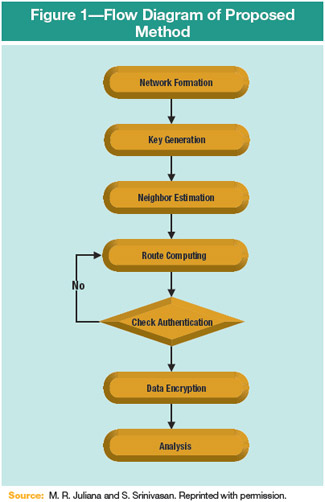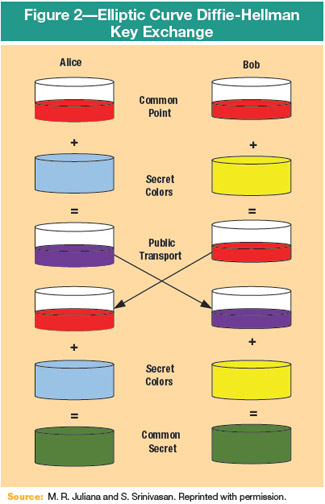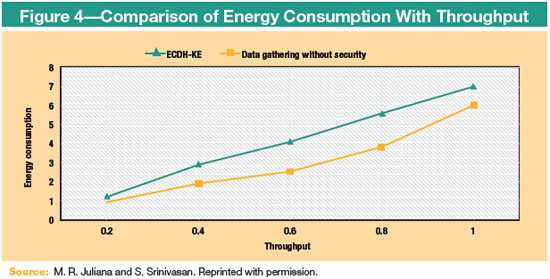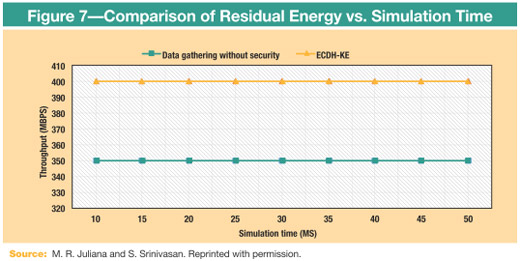# A Secure Data-gathering Approach in Wireless Sensor Networks: Considering QoS Via Hashing MechanismAuthor: Michael Roseline Juliana and Subramaniam Srinivasan, Ph.D.
Date Published: 1, May 2016

Data collection is a challenging task in wireless sensor networks (WSNs) due to the limitations in communication bandwidth and the energy budget.1 Many practical applications require continuous long-term data collection, without interruption for months or even years. Generally, WSNs consist of some number of battery-powered sensors. Through a multihop path, a sensor node transmits the information wirelessly to a receiver node with a limited communication range. Here, the multi-hop represents the communication between two end nodes via a number of intermediate nodes. Therefore, a single communication contains multiple paths to transmit the information. An efficient data-collection strategy is designed to minimize the energy cost of the sensor nodes; it also improves the network lifetime. In many applications, the gathering of continuous datasets from a resource-constrained WSN is unnecessary and difficult. It causes serious problems during the transmission of large amounts of data to the sink node. Due to the limited bandwidth of sensor nodes, packet drop reduces the quality of data. The largest amount of energy is consumed when more data are collected because data are transmitted or collected in the form of packets. In general, 0.1 J of energy is allocated for each and every packet; therefore, if more data are collected, obviously, a large amount of energy is consumed.

Secure communication is the most essential task to ensure the integrity and authenticity of transmitted data. In many applications, secure data transfer between the sensor nodes and the base station is also essential.2 While transferring the message, the base station must ensure that the obtained message should not be modified. A lightweight authentication scheme was required to protect data from unprivileged users, which is used in various WSN applications, e.g., military domains and health care monitoring. Generally, the multihop path becomes the target of attacks. It attacks nodes physically and creates a traffic collision or makes communication jam on the channel by generating radio interferences. Data encryption is essential in sensor networks when the sensors can be the subject of many types of attacks. Attackers can easily monitor and inject false data when the data are transmitted without encryption in the network.3 In general, sensor nodes encrypt the data on a hop-by-hop basis. An intermediate node keeps the keys of all sensing nodes, decrypts the received encrypted value and gathers all of the received values. Finally, the result in transmission to the base station is encrypted. This method is complicated and expensive due to the received data being decrypted before aggregation. Additionally, it produces an overhead imposed by key management.

To overcome these issues, this article focuses on a secure data-gathering scheme that considers throughput, delay and energy quality of service (QoS) parameters. To reduce the computational overhead of sensor nodes, this article proposes a new hash-based authentication scheme for WSNs that produces a strong and unique message authentication code for a particular message. A preshared secret key is obtained from the Elliptic Curve Diffie-Hellman Key Exchange (ECDH-KE) algorithm. This algorithm is designed based on a modified hash function that is used to calculate the message authentication code for giving messages. This algorithm delivers both integrity and the authenticity of a message with a single hash value. Before transmitting the message, the signature is verified by each sensor node to minimize the overhead introduced in the network.

## Using ECDH-KE

Suppose that Alice wants to transmit data to Bob. Initially, the network is formed by generating a private key for all nodes. After that, a neighbor estimation is done using Euclidean distance. To discover the route, the distance of the node from the source, Alice, to the destination, Bob, is calculated. The formula for finding the distance is:

D = v (a2 – a1)2 + (b2 – b1)2

Where (a1, b1) are the positions of the source node and (a2, b2) are the positions of the node from which the distance is calculated. After the distance is calculated, the route is computed. For authentication, the ECDH-KE formula is used, as it is a dependable algorithm in terms of communication, overhead limitations and energy consumption of the WSN. The ECDH-KE algorithm requires a preshared secret key to be used between sensor nodes (SNs) and the base station (BS). Between the BS and SN, a secret key (Sk) is proposed. The process is illustrated in figure 1.For transmitting messages, the authenticity and integrity must be easy and secure to calculate. A modified secure hash algorithm is used to compute the message authentication code of a given message, M. Using a regularly distributed pseudorandom function, a modified hash function is proposed. The default secure hash function uses the following logical functions in the main loop:

fp (X,Y,Z) = (X^Y) v ((~X)^Z)
fp (X,Y,Z) = X+Y+Z
fp (X,Y,Z) = (X^Y) V (X^Y) V (Y^Z)
fp (X,Y,Z) = X ? Y ? Z

With the help of the pseudorandom function, the previous logical functions are modified. Due to its randomness and lack of a repeating period, unique hash values are obtained for each message. The modified pseudorandom function with a secret key is defined as:

F (Qp ) = Qp * v2 *Sk

The previous equation is used as a message integrity and authenticity code. Based on the input message and secret key, the output value of the hash function is obtained. The appropriate hash value for the message can be computed by holders of the secret key only. Figure 2 uses color to show the simple model of key exchange.

Alice and Bob have kept their private keys (represented in figure 2 as their color) securely to themselves and have sent their public keys directly to each other. They fix a finite field, Ff, an elliptic curve, Ec, which was defined over the finite field, and base point B?Ec. Alice selects a random a?Ff, which keeps the key secret. It then computes the public key aB?Ec and transmits it to Bob. On the other side, Bob selects a random integer, b, and computes bB, which is transmitted to Alice. The common secret key is ?Ec.

An elliptic curve over a field is defined as:

y2+a1 xy+a3 y=x3+a2 x2+a4 x+a6

For any cryptographic technique, there is an analog for the elliptic curve. The ECDH-KE is one of the systems. In the proposed method, the encryption of the message is done by the Diffie-Hellman exchanging key. In encryption, the sender calculates the multiplication between the coordinates of the key.

## Algorithm 1: ECDH-KE

• Step 1: Alice and Bob select a finite field, F_f, and an elliptic curve, E_(c,), defined over it, E_c (F_f).
• Step 2: Both publicly choose a random base point, B, belonging in Ec.
• Step 3: Alice selects a secret random integer, n. Then she calculates nB?Ec and forwards it to Bob.
• Step 4: Bob selects a secret random integer, m. Then he calculates mB?Ec and forwards it to Alice.
• Step 5: nB and mB are public keys and n and m are secret keys.
• Step 6: Alice calculates the secret key, nmB=n(mB).
• Step 7: Bob calculates the secret key, nmB=m(nB).

## Performance Analysis

This section discusses the performance evaluation of the proposed ECDH-KE formula. The security-based data-gathering ECDH-KE method is compared with the existing data-gathering method that does not have security. The criteria of packet drop, energy consumption, network lifetime, residual energy and throughput are used for analyzing the performance.

Figure 3 shows the comparison graph for the number of packets dropped against simulation time. The execution time varies from 10 to 80 milliseconds. When more than one packet of data fails to reach its destination during transmission, packet drop occurs. When compared to the existing method, the ECDH-KE method results in fewer packet drops.The amount of energy consumption for the amount of work that can be performed (throughput) is plotted in figure 4. Energy is measured in joules, and throughput is measured in megabits per second (Mbps). The energy consumption of the ECDH-KE method is compared with the existing method. As seen in figure 4, the proposed method achieves significant energy savings in comparison to the existing method.

The lifetime of the network for the ECDH-KE method compared with the existing method is shown in figure 5. The graph considers the number of active nodes and compares that to the number of iterations in a network. The residual energy analysis of the proposed ECDH-KE method and existing method is depicted in figure 6.Figure 7 shows the throughput comparison graph for ECDH-KE and data gathering without a security method. The output performance shows that the proposed method provides significantly more throughput than the existing method.

## Conclusion

This article proposes an ECDH-KE algorithm to provide a security-based data-gathering approach. A preshared secret key exchange is used between the sensor nodes and base station, and it provides better security for data gathering. The transmitter computes the product between the coordinates of the key in the encryption algorithm. The experimental results show the effectiveness of ECDH-KE in terms of network lifetime, energy consumption and throughput, as compared to the existing method. Most of the existing research methodologies construct the secure data-gathering approach in WSN. However, there is one more issue that is important for data gathering, which is the consumption of energy. For future enhancement, this proposed methodology can be extended to reach low energy consumption through data gathering in a WSN.

## Endnotes

1 Wang, F.; J. Liu; “Networked Wireless Sensor Data Collection: Issues, Challenges, and Approaches,” IEEE Communications Surveys & Tutorials, vol. 13, June 2010, http://ieeexplore.ieee.org/xpl/articleDetails.jsp?arnumber=5497857
2 Shu, T; et al., “Secure Data Collection in Wireless Sensor Networks Using Randomized Dispersive Routes,” IEEE Transactions on Mobile Computing, vol. 9, July 2010, www.computer.org/csdl/trans/tm/2010/07/ttm2010070941-abs.html
3 Bahi, J.; et al., “Secure Data Aggregation in Wireless Sensor Networks: Homomorphism versus Watermarking Approach,” Ad Hoc Networks, vol. 49, edited by J. Zheng, et al., Eds., Springer Berlin Heidelberg, 2010, p. 344-358

Michael Roseline Juliana
Is an associate professor in the Department of Electronics and Communication Engineering at St. Michael College of Engineering and Technology (Kalayarkoil, Tamilnadu, India).

Subramaniam Srinivasan, Ph.D.
Is professor and head of the Department of Computer Science and Engineering at Anna University at the regional office in Madurai, Tamilnadu, India. He has published more than 90 research papers in journals, conferences and workshops.